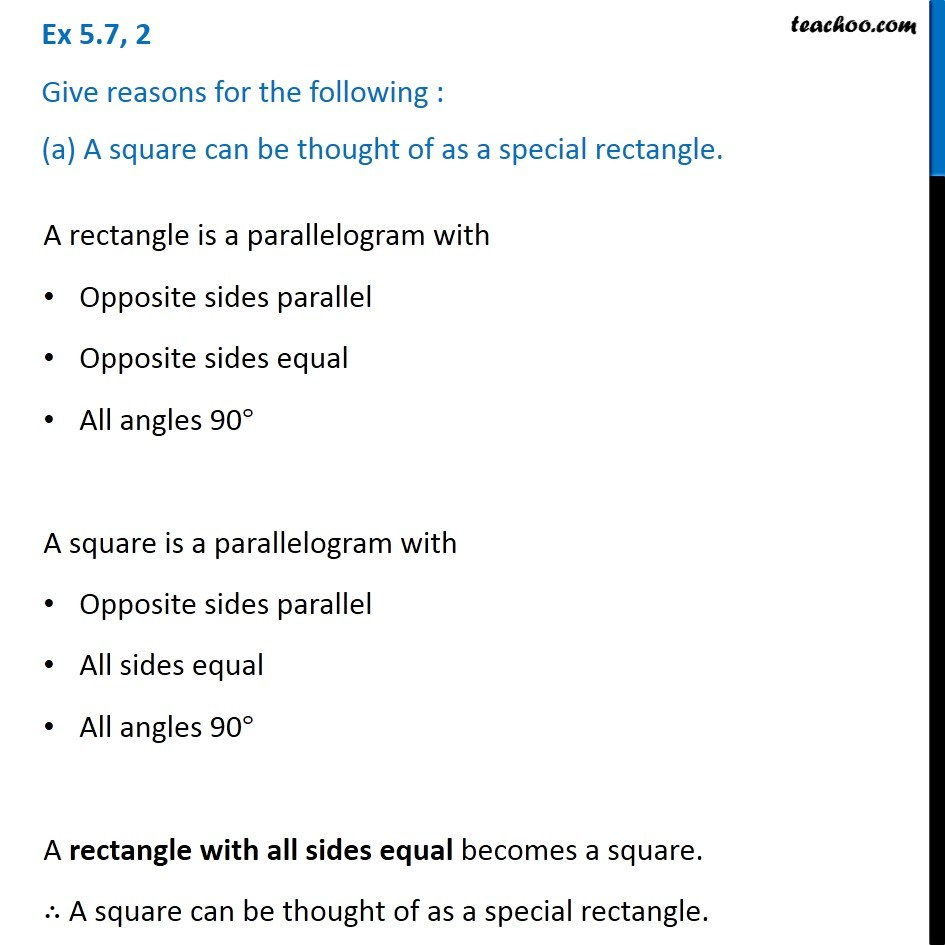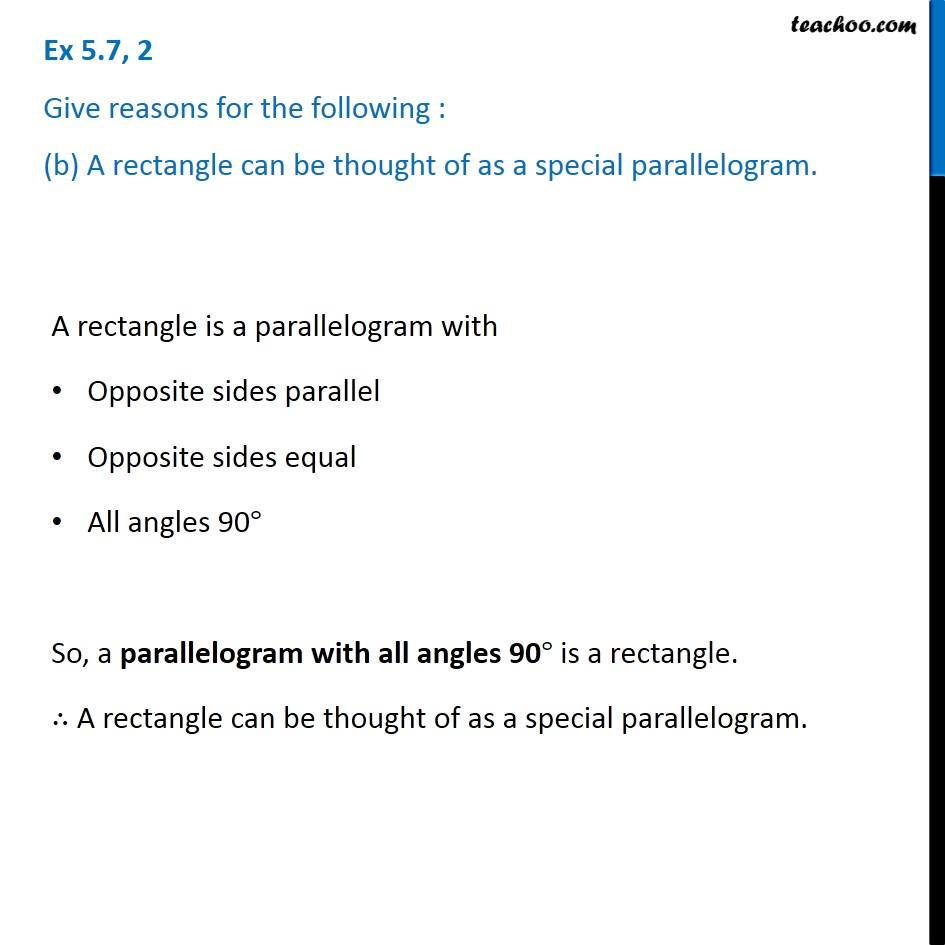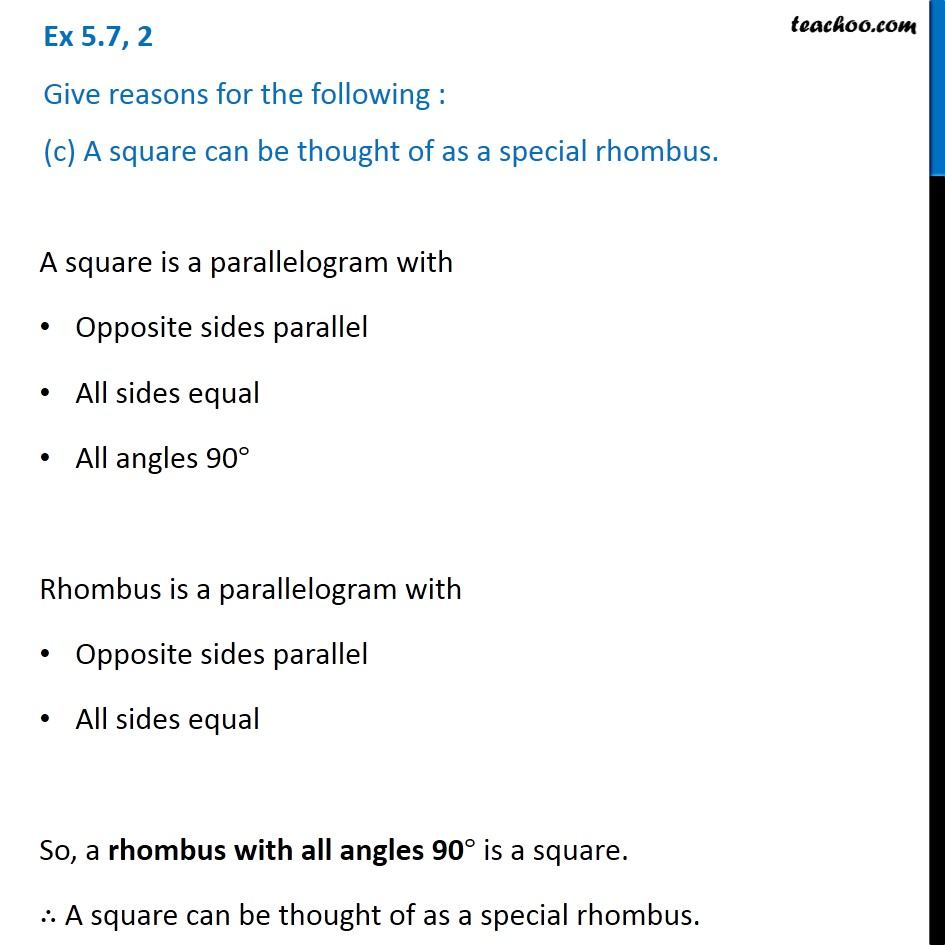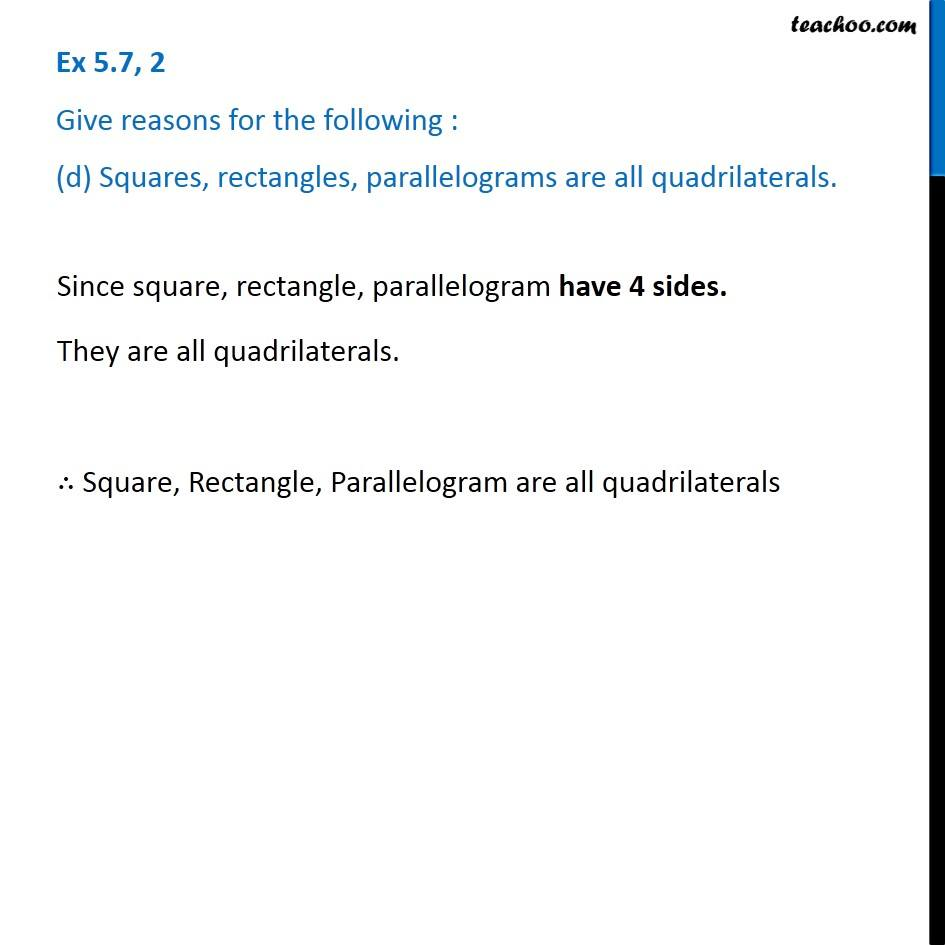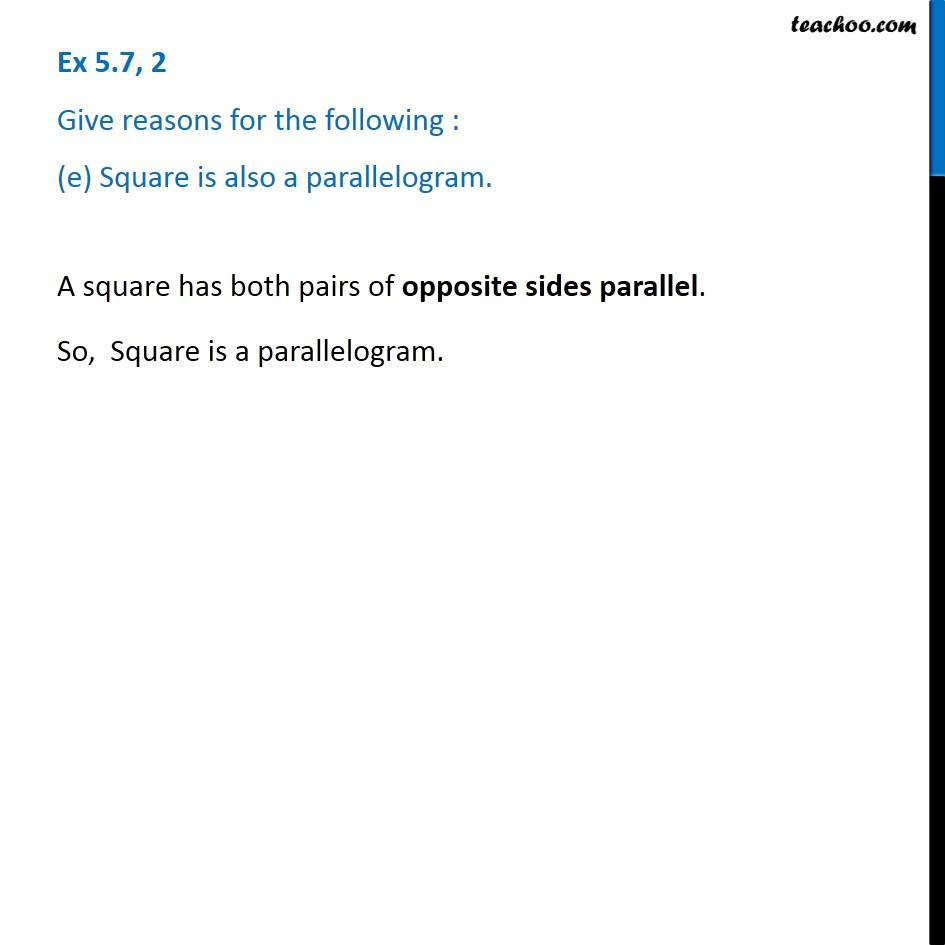Subscribe to our Youtube Channel - https://you.tube/teachoo

1. Chapter 5 Class 6 Understanding Elementary Shapes
2. Serial order wise
3. Ex 5.7

Transcript

Ex 5.7, 2 Give reasons for the following : (a) A square can be thought of as a special rectangle. A rectangle is a parallelogram with Opposite sides parallel Opposite sides equal All angles 90° A square is a parallelogram with Opposite sides parallel All sides equal All angles 90° A rectangle with all sides equal becomes a square. ∴ A square can be thought of as a special rectangle. Ex 5.7, 2 Give reasons for the following : (b) A rectangle can be thought of as a special parallelogram. A rectangle is a parallelogram with Opposite sides parallel Opposite sides equal All angles 90° So, a parallelogram with all angles 90° is a rectangle. ∴ A rectangle can be thought of as a special parallelogram. Ex 5.7, 2 Give reasons for the following : (c) A square can be thought of as a special rhombus. A square is a parallelogram with Opposite sides parallel All sides equal All angles 90° Rhombus is a parallelogram with Opposite sides parallel All sides equal So, a rhombus with all angles 90° is a square. ∴ A square can be thought of as a special rhombus. Ex 5.7, 2 Give reasons for the following : (d) Squares, rectangles, parallelograms are all quadrilaterals. Since square, rectangle, parallelogram have 4 sides. They are all quadrilaterals. ∴ Square, Rectangle, Parallelogram are all quadrilaterals Ex 5.7, 2 Give reasons for the following : (e) Square is also a parallelogram. A square has both pairs of opposite sides parallel. So, Square is a parallelogram.

Ex 5.7